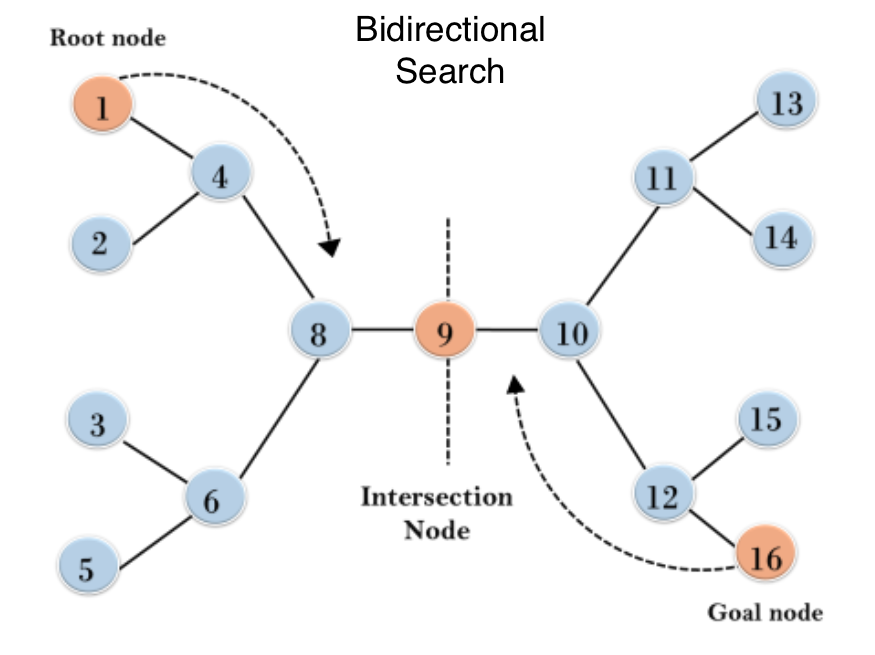Related Tags

python
twcompetition
communitycreator

# How to use bidirectional search implementation in Python

Ethel Esenam Klu

A graph is a network of nodes connected by arcs or edges.

The two basic graph search algorithms, Breadth-First SearchBFS and Depth-First SearchDFS aim to find a path between 2 nodes (preferably the shortest) and determine cycles in the graph.

A graph search is done in one direction, either from the source/initial vertex to the goal/target vertex.

To search from both ends simultaneously, a bidirectional search is implemented.

## Bidirectional search

Bidirectional search is a graph search algorithm that finds the shortest path from a source vertex to a goal vertex.

In implementing bidirectional search in Python, the graph search can either be:

1. Forward search from the source to the goal vertex.
2. Backward search from the goal to the source vertex.

The intersection of both forward and backward graphs indicates the end of the search, as displayed below.1. Less time consuming as the shortest path length is used for the search. The goal node has to be known before the search can be done simultaneously in both directions.

#### When is it possible to use bidirectional search in Python?

The bidirectional approach can be used:

1. When the branching factor for the forward and reverse direction is the same.
2. When both the source and goal vertices are specified and distinct from each other.
##### Bidirectional search implementation in Python

Below is an implementation of bidirectional search in Python using BFS:



def __init__(self, v):

self.vertex = v
self.next = None

class bidirectional_Search:

def __init__(self, vertices):

self.vertices = vertices
self.graph = [None] * self.vertices

self.source_queue = list()
self.last_node_queue = list()

self.source_visited = [False] * self.vertices
self.last_node_visited = [False] * self.vertices

self.source_parent = [None] * self.vertices
self.last_node_parent = [None] * self.vertices

node.next = self.graph[source]
self.graph[source] = node

node.next = self.graph[last_node]
self.graph[last_node] = node

if direction == 'forward':

current = self.source_queue.pop(0)
connected_node = self.graph[current]

while connected_node:
vertex = connected_node.vertex

if not self.source_visited[vertex]:
self.source_queue.append(vertex)
self.source_visited[vertex] = True
self.source_parent[vertex] = current

connected_node = connected_node.next
else:

current = self.last_node_queue.pop(0)
connected_node = self.graph[current]

while connected_node:
vertex = connected_node.vertex

if not self.last_node_visited[vertex]:
self.last_node_queue.append(vertex)
self.last_node_visited[vertex] = True
self.last_node_parent[vertex] = current

connected_node = connected_node.next

def is_intersecting(self):

#
for i in range(self.vertices):
if (self.source_visited[i] and
self.last_node_visited[i]):
return i

return -1

def path_st(self, intersecting_node,
source, last_node):

path = list()
path.append(intersecting_node)
i = intersecting_node

while i != source:
path.append(self.source_parent[i])
i = self.source_parent[i]

path = path[::-1]
i = intersecting_node

while i != last_node:
path.append(self.last_node_parent[i])
i = self.last_node_parent[i]

print("*****Path*****")
path = list(map(str, path))

print(' '.join(path))

def bidirectional_search(self, source, last_node):

self.source_queue.append(source)
self.source_visited[source] = True
self.source_parent[source] = -1

self.last_node_queue.append(last_node)
self.last_node_visited[last_node] = True
self.last_node_parent[last_node] = -1

while self.source_queue and self.last_node_queue:

intersecting_node = self.is_intersecting()

if intersecting_node != -1:
print("Path exists between {} and {}".format(source, last_node))
print("Intersection at : {}".format(intersecting_node))
self.path_st(intersecting_node,
source, last_node)
exit(0)
return -1

if __name__ == '__main__':

n = 17

source = 1

last_node = 16

my_Graph = bidirectional_Search(n)

out = my_Graph.bidirectional_search(source, last_node)

if out == -1:
print("No path between {} and {}".format(source, last_node))


RELATED TAGS

python
twcompetition
communitycreator

CONTRIBUTOR

Ethel Esenam Klu
RELATED COURSES

View all Courses

Keep Exploring

Learn in-demand tech skills in half the time